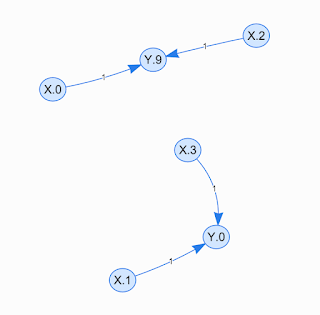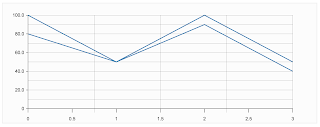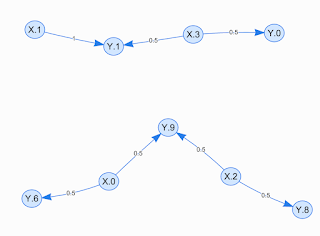## Monday 1 February 2016

### A tool to build Bayesian Network from a time series

I showed in the last post (How to convert "Time Series" to "Bayesian Network") how we can convert a sample time series to a Bayesian Network as you may have seen it is easy, but there are some things we have to talk about them a bit more.

1- You do not need to be worried about the maximum value of the Y axis. Whatever it is 10, 1000, 100K, 10G, ... your resolution of processing depends on the overall form of the series you are looking or processing, not these measures or units. What I mean is that a series that shows a network traffic with a maximum of 10G in one day can be considered a series showing the body temperature of a human! We don't care what it is about or in what unit is its Y or X (time) axes.

2- Like the Y axis, the length of X axis or resolution of time-period doesn't matter too, an hour, a day, a month, whatever it is, we only deal with some low-resolution measurement for this axis. For example, in our daily network traffic, we may choose a resolution of an hour to build our time or X nodes. So we only have 24 nodes of type time, each representing a specific hour in a day.

3- What is crucial and builds the pattern is the edges, the relation between nodes. You have for example 10 different Y nodes and 24 different X (or Time) nodes. If your body temperature goes down at midnight when you are sleeping, the Bayesian Network should just keep having some high probability of lower temperature during the night nodes and low probability for higher temperature, what could be normal with high probability when the subject is awake.

Now the question is what happens if we use more than one period of pattern to build our Bayesian Network?
First note that using one period of your time series to construct a network is shaping your system based on the given period, you can call it the learning process. It is like looking or observing a cat for the first time. The effect of feeding more than one period of data to the Bayesian Network is exactly like looking and observing some other cats to know a cat better. It helps the model learns the existing pattern better, or in our cat example if you observe many cats after awhile you can easily distinguish cats from other animals or find any abnormal feature in them.Simple single period [100,50,100,50] time series.

Let us have a very simple example, consider you have single period of a time series like 100,50,100,50 you can see how it looks like in the provided tool here 100,50,100,50Network graph of single period [100,50,100,50]  time series.

Now consider systems usually are not that much exact so next time you may face your system shows this series 80,50,90,40Two periods of single time series.

What the second Bayesian Network shows is that for example at X=0 you have two choices, either having Y=6 or Y=9 while in X=1 you only can have Y=1, these are also visible from the X-Y graph.Bayesian Network of two periods of  single time series.

Now try this one which shows a simple time shift which usually happens in time series, the resulting network learns that the maximum which is Y=9, sometimes happens at X=6 and sometimes at X=7.Sample two periods of time series with time shift.

The following graph is the Bayesian Network representation for the above two periods.Bayesian Network of the time series with time shift.

Now finally, you can test your time series patterns in the provided tool; it is not a  professional one, but it works pretty well with positive numbers.

Check it out yourself: Simple time series analysis